# How to sort a collection in JavaScript ?

A javascript collection is much like a container.  It’s just an item that combines several elements into a single unit. Aggregate information is stored, accessed, modified, and communicated via collections.

With the help of constructors, we create collections in javascript. In earlier versions of javascript constructors are expressed as functions and utilized in the same way. under collections come arrays, sets, and maps. Let’s learn how to sort them in this article.

We use the JavaScript sort() method to sort collections. This method sorts the array in place.

Syntax:

`Array.prototype.sort()`

SORTING AN ARRAY

Example 1: Sorting an array when the array has numbers as elements:

## HTML

 ` ` `<``html``> ` ` `  `<``head``> ` `    ``<``title``>Javascript sorting collections ` ` ` ` `  `<``body``> ` `    ``<``script``> ` `        ``// Sorting an array in ascending order ` `        ``let array = [10, 2, 5, 12, 7]; ` `        ``array = array.sort(function (a, b) { ` `            ``return a - b; ` `        ``}); ` `        ``console.log("sorted array : " + array); ` `    `` ` ` ` ` `  ``

Output: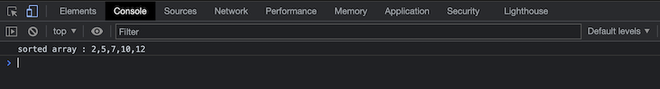Example 2: Sorting an array when array consists of strings:

## HTML

 ` ` `<``html``> ` ` `  `<``head``> ` `    ``<``title``>Javascript sorting collections ` ` ` ` `  `<``body``> ` `    ``<``script``> ` `        ``// Sorting an array of strings ` `        ``let array = ["c", "b", "a"]; ` `        ``array = array.sort(); ` `        ``console.log("sorted array : " + array); ` ` `  `    `` ` ` ` ` `  ``

Output: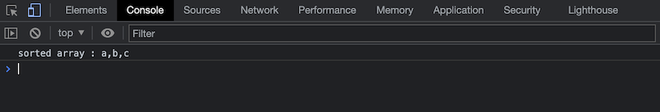SORTING A MAP

Sorting a map when:

• Maps have pairs of keys and values.
• Maps work like dictionaries.
• We’ve to convert the Map to Array to sort.

Example 1: Sorting according to values:

## HTML

 ` ` `<``html``> ` ` `  `<``head``> ` `    ``<``title``>Javascript sorting collections ` ` ` ` `  `<``body``> ` `    ``<``script``> ` ` `  `        ``// Creating a map and sorting it according to values. ` `        ``let newMap = new Map(); ` `        ``newMap.set("a", 50); ` `        ``newMap.set("c", 40); ` `        ``newMap.set("b", 30); ` `        ``newMap.set("d", 10); ` `        ``newMap = Array.from(newMap).sort((a, b) => a - b); ` `        ``console.log(newMap); ` `    `` ` ` ` ` `  ``

Output: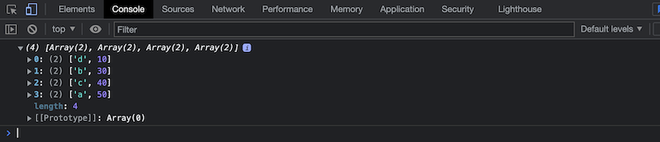Example 2: Sorting according to keys:

## HTML

 ` ` `<``html``> ` ` `  `<``head``> ` `    ``<``title``>Javascript sorting collections ` ` ` ` `  `<``body``> ` `    ``<``script``> ` `        ``// Sorting map by keys ` `        ``let newMap = new Map(); ` `        ``newMap.set("a", 50); ` `        ``newMap.set("c", 40); ` `        ``newMap.set("b", 30); ` `        ``newMap.set("d", 10); ` `        ``newMap = Array.from(newMap.entries()).sort(); ` `        ``console.log(newMap); ` `    `` ` ` ` ` `  ``

Output: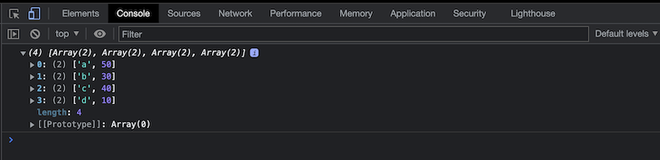SORTING A SET

Sorting a set when:

• We create a new set using the new keyword.
• The set contains duplicate values which are removed after the code is run.
• We need to convert the set created to Array in order to sort it.

Example 1: Sorting a set that contains numbers:

## HTML

 ` ` `<``html``> ` ` `  `<``head``> ` `    ``<``title``>Javascript sorting collections ` ` ` ` `  `<``body``> ` `    ``<``script``> ` `        ``// Converting a set into an array and sorting it. ` `        ``new_set = Array.from(new Set([9, 9, 2, 4, 5, 11])) ` `          ``.sort(function (a, b) { ` `            ``return a - b; ` `        ``}); ` `        ``console.log(new_set); ` `    `` ` ` ` ` `  ``

Output: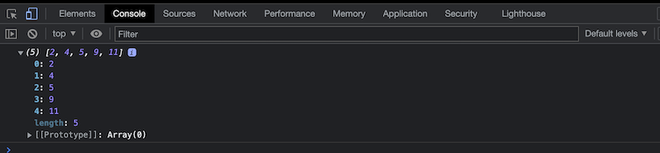Example 2: Sorting a set of strings:

## HTML

 ` ` `<``html``> ` ` `  `<``head``> ` `    ``<``title``>Javascript sorting collections ` ` ` ` `  `<``body``> ` `    ``<``script``> ` `        ``new_set = Array.from(new Set(["rachel", "sam", "daniel"])) ` `          ``.sort(); ` `        ``console.log(new_set); ` `    `` ` ` ` ` `  ``

Output: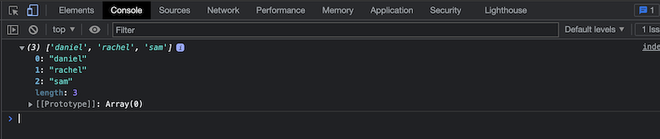Whether you're preparing for your first job interview or aiming to upskill in this ever-evolving tech landscape, GeeksforGeeks Courses are your key to success. We provide top-quality content at affordable prices, all geared towards accelerating your growth in a time-bound manner. Join the millions we've already empowered, and we're here to do the same for you. Don't miss out - check it out now!

Previous
Next